# Orthogonal System of Functions

The following article is from The Great Soviet Encyclopedia (1979). It might be outdated or ideologically biased.

## Orthogonal System of Functions

a system of functions {ϕn(x)}, n = 1, 2, …, that are orthogonal with respect to some weight function ρ(x) on the interval [a, b]; that is.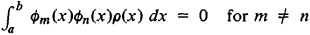For example, the trigonometric system 1, cos nx, sin nx, for n = 1, 2, …, is an orthogonal system with weight 1 on the interval [–π, π]. The Bessel functions Jv(μnvxl), where n = 1, 2,…, and the μnv are the positive zeros of Jv (x), form for each v > –½ an orthogonal system with weight x on the interval [0, l].

If every function ϕ (x) of an orthogonal system of functions satisfies the condition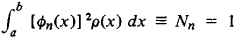then the system of functions is said to be normalized. Any orthogonal system of functions can be normalized by multiplying ϕn(x) by the normalizing factor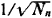.

The systematic study of orthogonal systems of functions began with Fourier’s method of solving boundary value problems in mathematical physics. This method yields, for example, solutions of the Sturm-Liouville problem for the equation [ρ(x)y′]′ + q(x)y = λy that satisfy the boundary conditions y(a) + hy′(a) = 0 and (b) + Hy′(b) = 0, where h and H are constants. These solutions, the eigenfunctions of the problem, form an orthogonal system of functions with weight ρ(x) on the interval [a, b].

In his research on interpolation by the method of least squares and on the problem of moments P. L. Chebyshev discovered an extremely important class of systems of orthogonal functions—the class of systems of orthogonal polynomials. In the 20th century, research on orthogonal systems of functions has been based chiefly on the theory of the Lebesgue integral and Lebesgue measure and has become an independent branch of mathematics. A fundamental problem of the theory of systems of orthogonal functions is the expansion of a function f(x) in a series of the form ∑Cnϕn(x), where {ϕn(x)} is an orthogonal system of functions. If we put formally f(x) = ∑Cnϕn (x), where {ϕn(x)} is a normalized system of orthogonal functions, and allow term-by-term integration, then by multiplying this series by ϕn (x)ρ(x) and integrating from a to b, we obtain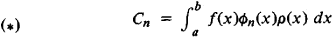The coefficients Cn, which are called the Fourier coefficients of the function with respect to the system {ϕn(x)}, have the property that the linear combination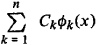best approximates this function in the mean. In other words, the mean square error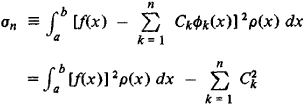has the smallest value compared with the value associated with any other linear combinatior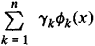for the same n. This fact implies, in particular, the Bessel inequality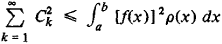The series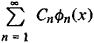where the coefficients Cn are computed from formula (*), is called the Fourier series of f(x) with respect to the orthonormal system {ϕn(x)}. Of prime importance for applications is the question of whether the function f(x) is uniquely determined by its Fourier coefficients. Orthogonal systems of functions for which this is the case are called complete. The conditions for the completeness of an orthogonal system of functions can be given in several equivalent forms. (1) Any continuous function f(x) can be approximated in the mean to any desired degree of accuracy by linear combinations of the functions ϕk (x); that is,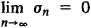The series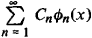is then said to converge in the mean to the function f(x). (2) For any function f(x) that is square integrable with respect to the weight p(x), the Liapunov-Steklov (Parseval) completeness condition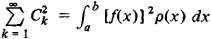is satisfied. (3) There does not exist a nonzero function that is square integrable on the interval [a, b] and is orthogonal to all the functions ϕn(x), n = 1, 2, ….

If square integrable functions are viewed as elements of a Hilbert space, then orthonormal systems of functions are systems of basis vectors for the space, and expansion in a series of orthonormal functions is the expansion of a vector in terms of the basis vectors. With this approach, many concepts of the theory of orthonormal systems of functions acquire an obvious geometric meaning. For example, formula (*) says that the projection of a vector on a basis vector is equal to the scalar product of the vector and that basis vector. Moreover, the Liapunov-Steklov (Parseval) equality can be interpreted as the Pythagoras theorem for an infinite-dimensional space—that is, the square of the length of a vector is equal to the sum of the squares of its projections on the coordinate axes. Completeness of an orthogonal system of functions means that the smallest complete sub-space containing all the vectors of the system coincides with the entire space. Other examples could easily be given.

### REFERENCES

Tolstov, G. P. Riady Fur’e, 2nd ed. Moscow, 1960.
Natanson, I. P. Konstruktivnaia teoriia funktsii. Moscow-Leningrad, 1949.
Natanson, I. P. Teoriia funktsii veshchestvennoi peremennoi, 2nd ed. Moscow, 1957.
Jackson, D. Riady Fur’e i ortogonal’nye polinomy. Moscow, 1948. (Translated from English.)
Kaczmarz, S., and H. Steinhaus. Teoriia ortogonal’nykh riadov. Moscow, 1958. (Translated from German.)